Question

For the following circuit, assume that R1 = 1 ohm, R2 = 2 ohms, R3 = 8 ohms, and R4 = 4 ohms. Also assume that V1 = 50 volts and V2 = 100 volts. Find the current through each of the resistors and the power dissipated through all of the resistors. (Hint: You’ll need to use Kirchoff’s Loop and Junction Rule)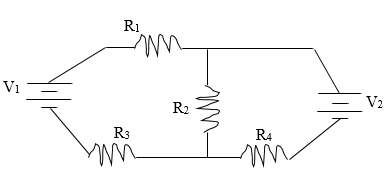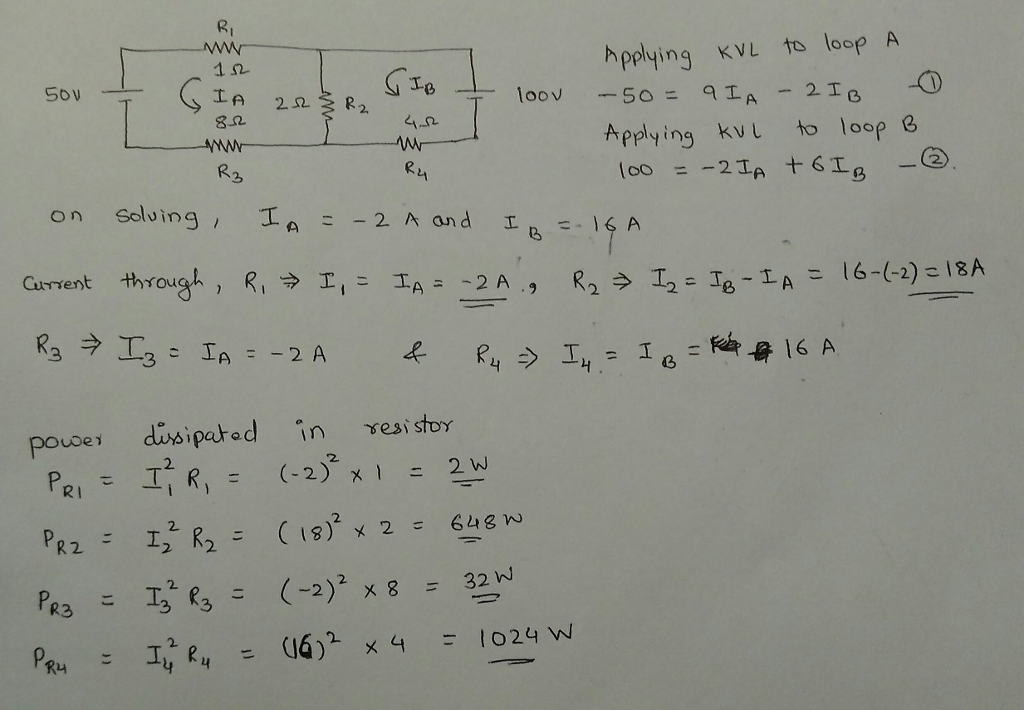kindly rate

#### Earn Coins

Coins can be redeemed for fabulous gifts.

Similar Homework Help Questions
• ### given the following circuit v= 66 volts R1= 5 ohms R2 = 14 ohms R3 =...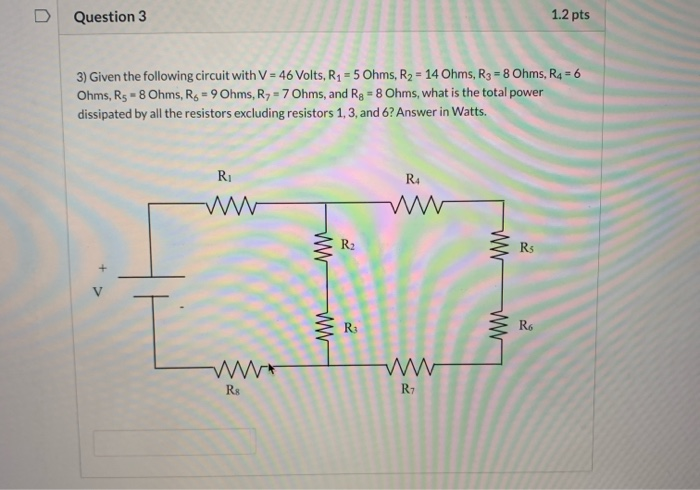given the following circuit v= 66 volts R1= 5 ohms R2 = 14 ohms R3 = 8 ohms R4 =6 ohms R5=8 ohms R6= 9 ohms R7= 7 ohms R8= 8 ohms what is the total power dissipated by all the resisitors excluding 1,3, and 6 answer in watts. Question 3 1.2 pts 3) Given the following circuit with V = 46 Volts, R2 = 5 Ohms, R2 = 14 Ohms, R3 = 8 Ohms, R = 6 Ohms, Rs...

• ### R1= 24.8 ohms R2= 13.9 ohms R3= 25.5 ohms V1= 5 volts V2= 11.9 volts V3=...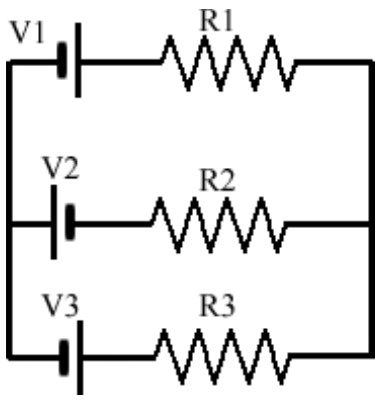R1= 24.8 ohms R2= 13.9 ohms R3= 25.5 ohms V1= 5 volts V2= 11.9 volts V3= 9.1 volts What is the current through the 13.9 ohm resistor? R1 V1 V2 R2 V3 R3

• ### In the circuit below given that R1 = 500 Ohm, R2 = 820 Ohm, R3 =...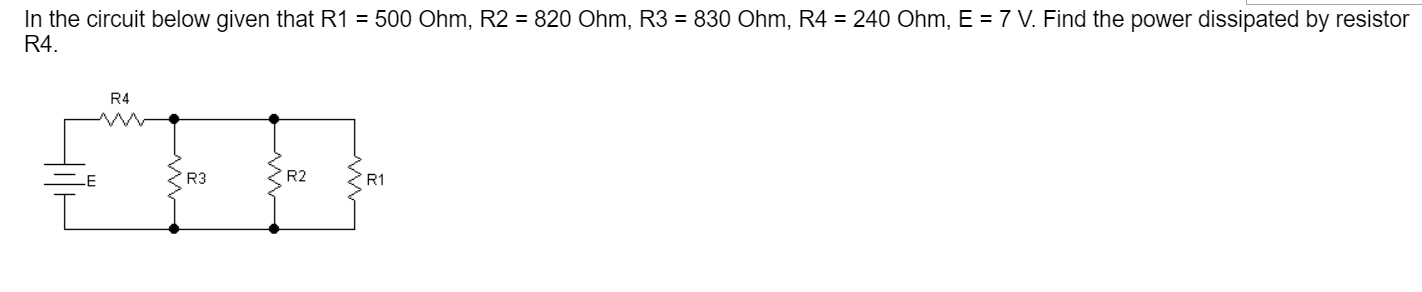In the circuit below given that R1 = 500 Ohm, R2 = 820 Ohm, R3 = 830 Ohm, R4 = 240 Ohm, E = 7 V. Find the power dissipated by resistor R4. R4 E R3 R2 R1

• ### In the circuit above, the battery has EMF of 12 V, R1 is 1 Ohm, R2 is 2 Ohms and R3 is 3 Ohms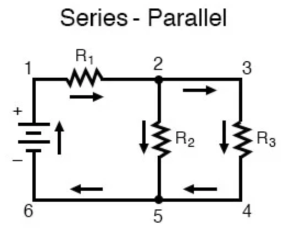In the circuit above, the battery has EMF of 12 V, R1 is 1 Ohm, R2 is 2 Ohms and R3 is 3 Ohms Find the power dissipated by R3Find the voltage drop across R1

• ### In the circuit, V1=129 V, V2=72 V, I0=2 A, R1=7 Ohm, R2=46 Ohm, R3=19 Ohm, R4=19...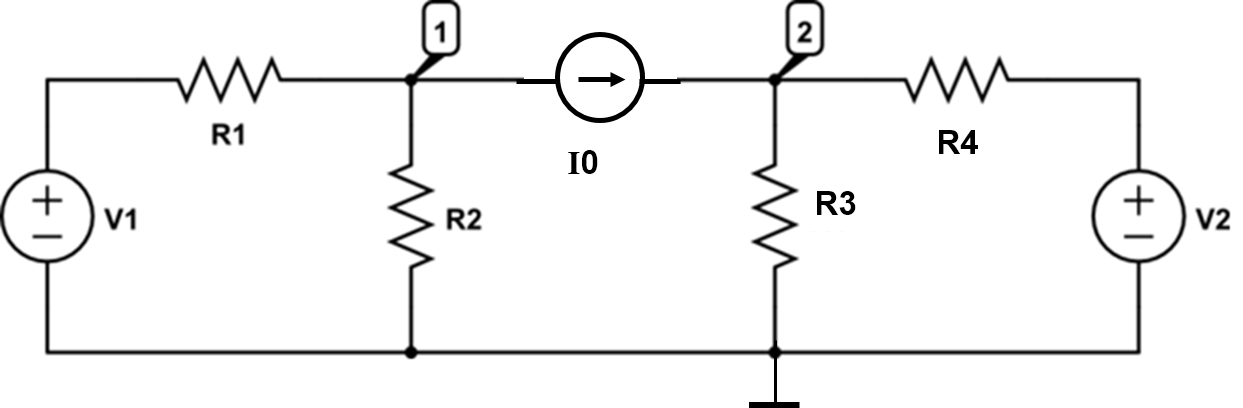In the circuit, V1=129 V, V2=72 V, I0=2 A, R1=7 Ohm, R2=46 Ohm, R3=19 Ohm, R4=19 Ohm. Solve the circuit using nodal analysis and answer the questions below. Find the current through R1 flowing in the direction from left to right. (Unit: A) Find the current through R2 flowing downwards. (Unit: A) Find the current through R3 flowing downwards. (Unit: A) Find the current thrrough R4 flowing from left to right. (Unit: A) R1 R4

• ### Assume the resistance values are R1 = 2,200 Ohm, R2 = 1,300 Ohm, R3 = 4,200...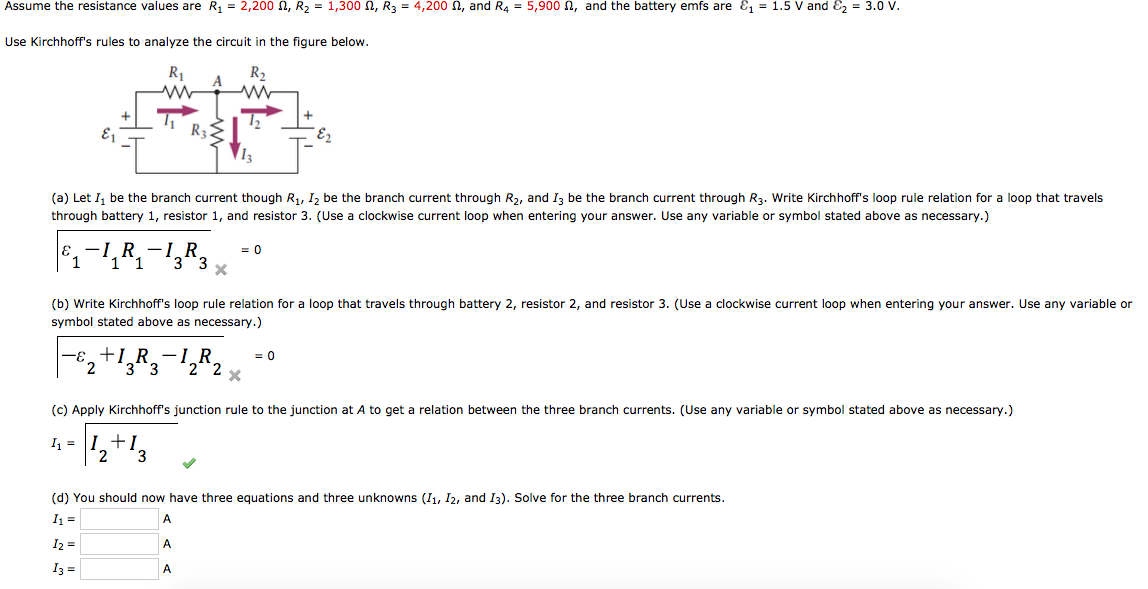Assume the resistance values are R1 = 2,200 Ohm, R2 = 1,300 Ohm, R3 = 4,200 Ohm, and R4 = 5,900 Ohm, and the battery emfs are epsilon1 = 1.5 V and Epsilon2 = 3.0 V. Use Kirchhoff?s rules to analyze the circuit in the figure below. (a) Let I1 be the branch current though R1, I2 be the branch current through R2, and I3 be the branch current through R3. Write Kirchhoff?s loop rule relation for a loop that...

• ### Consider the circuit shown below: R1 = 600 ohm R2 = 480 ohm R3 = 240...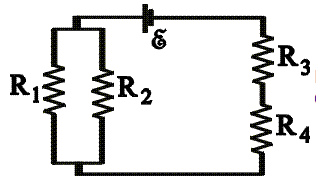Consider the circuit shown below: R1 = 600 ohm R2 = 480 ohm R3 = 240 ohm R4 = 210 ohm ε = 4.5 V a)Calculate the potential difference across R4. b)Calculate the power dissipated in R3. AA CN.

• ### Consider a circuit with three resistors (R1 = 40.5 ohms, R2 = 54.8 ohms, and R3...

Consider a circuit with three resistors (R1 = 40.5 ohms, R2 = 54.8 ohms, and R3 = 30.8 ohms) connected to a 12.5 V battery as follows: R1 and R2 are connected in parallel. This combination is then connected in series to R3. Find the power dissipated in R1. Calculate the answer in watts (W) and rounded to three significant figures. please explain.

• ### R2 R1 R3 R4 E In the following circuit, R1=0.8 4 Ohm, R2-2' 4 Ohm, R3-3'...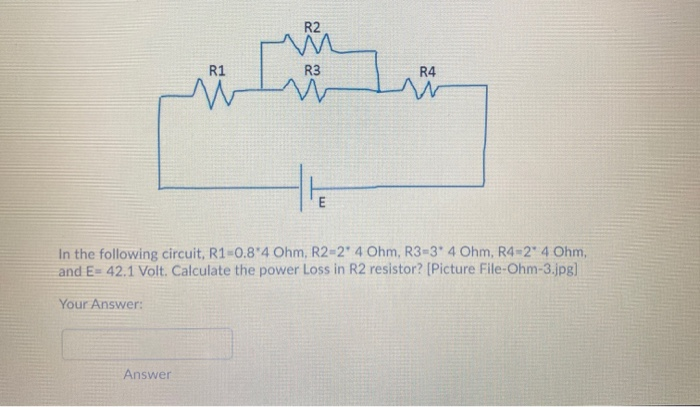R2 R1 R3 R4 E In the following circuit, R1=0.8 4 Ohm, R2-2' 4 Ohm, R3-3' 4 Ohm, R4-2° 4 Ohm, and E= 42.1 Volt. Calculate the power Loss in R2 resistor? [Picture File-Ohm-3.jpg] Your Answer: Answer

• ### R1 R3 R2 R4 W The circuit in the diagram contains one battery and four resistors,...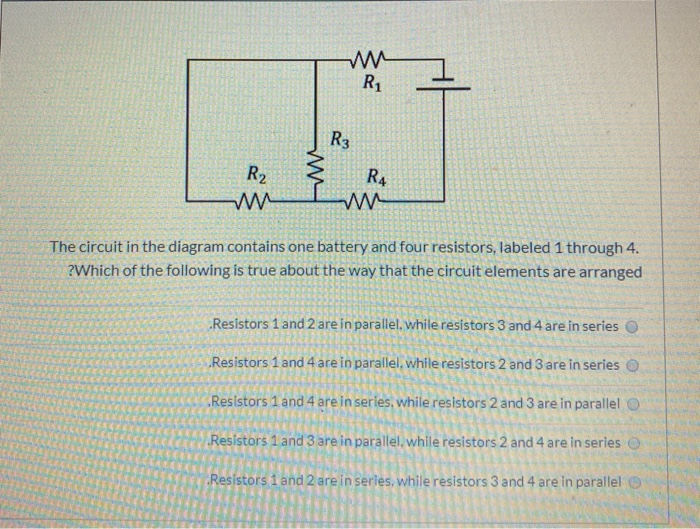R1 R3 R2 R4 W The circuit in the diagram contains one battery and four resistors, labeled 1 through 4. ?Which of the following is true about the way that the circuit elements are arranged Resistors 1 and 2 are in parallel while resistors 3 and 4 are in series Resistors 1 and 4 are in parallel while resistors 2 and 3 are in series Resistors 1 and 4 are in series, while resistors 2 and 3 are in parallel...